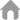您当前的位置：首页 > Java培训 > JAVA开发 >

java Random怎么用

Random类 (java.util)

Random类中实现的随机算法是伪随机，也就是有规则的随机。在进行随机时，随机算法的起源数字称为种子数(seed)，在种子数的基础上进行一定的变换，从而产生需要的随机数字。1、Random对象的生成

Random类包含两个构造方法，下面依次进行介绍：

a、public Random()

b、public Random(long seed)

Random r = new Random();

Random r1 = new Random(10);

2、Random类中的常用方法

Random类中的方法比较简单，每个方法的功能也很容易理解。需要说明的是，Random类中各方法生成的随机数字都是均匀分布的，也就是说区间内部的数字生成的几率是均等的。下面对这些方法做一下基本的介绍：

a、public boolean nextBoolean()

b、public double nextDouble()

c、public int nextInt()

d、public int nextInt(int n)

e、public void setSeed(long seed)

3、Random类使用示例

Random r = new Random();

a、生成[0,1.0)区间的小数

double d1 = r.nextDouble();

b、生成[0,5.0)区间的小数

double d2 = r.nextDouble() * 5;

c、生成[1,2.5)区间的小数

double d3 = r.nextDouble() * 1.5 + 1;

d、生成任意整数

int n1 = r.nextInt();

e、生成[0,10)区间的整数

int n2 = r.nextInt(10);

n2 = Math.abs(r.nextInt() % 10);

int n2 = r.nextInt(n);

n2 = Math.abs(r.nextInt() % n);

f、生成[0,10]区间的整数

int n3 = r.nextInt(11);

n3 = Math.abs(r.nextInt() % 11);

g、生成[-3,15)区间的整数

int n4 = r.nextInt(18) - 3;

n4 = Math.abs(r.nextInt() % 18) - 3;

h、几率实现

int n5 = r.nextInt(100);

int m; //结果数字

if(n5 < 55){ //55个数字的区间，55%的几率

m = 1;

}else if(n5 < 95){//[55,95)，40个数字的区间，40%的几率

m = 2;

}else{

m = 3;

}

int n6 = r.nextInt(20);

int m1;

if(n6 < 11){

m1 = 1;

}else if(n6 < 19){

m1= 2;

}else{

m1 = 3;

}

4、其它问题

a、相同种子数Random对象问题

Random r1 = new Random(10);

Random r2 = new Random(10);

for(int i = 0;i < 2;i++){

System.out.println(r1.nextInt());

System.out.println(r2.nextInt());

}

b、关于Math类中的random方法400-611-6270

Copyright ©2004-2018 华清远见 版权所有